# Unit conversion - high school - math problems

#### Number of problems found: 148

• RectangleThe rectangle is 21 cm long and 38 cm wide. Determine the radius of the circle circumscribing rectangle.
• Power line poleFrom point A, the power line pole is seen at an angle of 18 degrees. From point B to which we get when going from point A 30m away from the column at an angle of 10 degrees. Find the height of the power pole.
• Cylinder horizontallyThe cylinder with a diameter of 3 m and a height/length of 15 m is laid horizontally. Water is poured into it, reaching a height of 60 cm below the axis of the cylinder. How many hectoliters of water is in the cylinder?
• Tetrahedral prism - rhomboid baseCalculate the area and volume tetrahedral prism that has base rhomboid shape and its dimensions are: a = 12 cm, b = 70 mm, v_a = 6 cm, v_h = 1 dm.
• The poolThe cube-shaped pool has 140 cubic meters of water. Determine the dimensions of the bottom if the depth of the water is 200 cm and one dimension of the bottom is 3 m greater than the other. What are the dimensions of the pool bottom?
• Circular poolThe base of the pool is a circle with a radius r = 10 m, excluding a circular segment that determines the chord length 10 meters. The pool depth is h = 2m. How many hectoliters of water can fit into the pool?
• Railway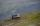Between points A, B, whose horizontal distance is 1.5 km railway line has 8promile climb. Between points B, C with horizontal distance of 900 m is climb 14promile. Calculate differences of altitudes between points A and C.
• Natural fertilizerThe rectangular garden measuring 120m and 60m was fertilized with 16kg of natural fertilizer. Natural fertilizer contains 45% organic matter. How much organic matter falls on 1 m2 of garden?
• Aircrafts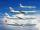Above the town hall tower flew the plane with constant speed 510 km/h and 15 minutes later the second plane at speed of 703 km/h. How long and how far from the town hall will be aircrafts caught up?
• Tower modelTower height is 300 meters, weight 8000 tons. How high is the model of the tower weight 1 kg? (State the result in the centimeters). The model is made from exactly the same material as the original no numbers need to be rounded. The result is a three-digi
• Car crashOn the road, with a maximum permitted speed of 60 km/h, there was a car crash. From the length of the vehicle's braking distance, which was 40 m, the police investigated whether the driver did not exceed that speed. What is the conclusion of the police, a
• Angles of elevationFrom points A and B on level ground, the angles of elevation of the top of a building are 25° and 37° respectively. If |AB| = 57m, calculate, to the nearest meter, the distances of the top of the building from A and B if they are both on the same side of
• The spacecraft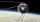The spacecraft spotted a radar device at altitude angle alpha = 34 degrees 37 minutes and had a distance of u = 615km from Earth's observation point. Calculate the distance d of the spacecraft from Earth at the moment of observation. Earth is considered a
• Roof 8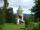How many liters of air are under the roof of tower which has the shape of a regular six-sided pyramid with a 3,6-meter-long bottom edge and a 2,5-meter height? Calculate the supporting columns occupy about 7% of the volume under the roof.
• Diagonals in the diamondThe length of one diagonal in diamond is 24 cm greater than the length of the second diagonal and diamond area is 50 m2. Determine the sizes of the diagonals.
• Two carsTwo cars (trucks and passenger) drove simultaneously from two places 50 km away. If they go against each other, they will meet in 30 minutes. If they follow each other in the same direction, the car will catch up with the truck in 2.5 hours. What is the s
• Dynamometer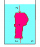What is the volume of a body that stretches a dynamometer in air, on which it is suspended by a force of 2.5 N, and if it is immersed in alcohol with a density of 800 kg/m ^ 3, does it tension the dynamometer with a force of 1.3 N?
• SSA and geometryThe distance between the points P and Q was 356 m measured in the terrain. The PQ line can be seen from the viewer at a viewing angle of 107° 22 '. The observer's distance from P is 271 m. Determine the viewing angle of P and observer.
• The cyclist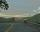The cyclist went from village to town. The first half of the journey went at 20 km/h. The second half of the journey, which mostly fell, went at 39 km/h. All journey took 88 minutes. Calculate the distance from the village to the town.
• Long hot dog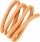In Kostelec above Black Forest, they made a record-long hot dog. When slowly moved it to the square, one of the spectators decided to measure its length: the size of his footprint is 28 cm; he measured 20 feet in travel direction, counted 5 feet against t

Do you have an interesting mathematical word problem that you can't solve it? Submit a math problem, and we can try to solve it.

We will send a solution to your e-mail address. Solved examples are also published here. Please enter the e-mail correctly and check whether you don't have a full mailbox.

Please do not submit problems from current active competitions such as Mathematical Olympiad, correspondence seminars etc...

Unit conversion - math problems. Examples for secondary school students.